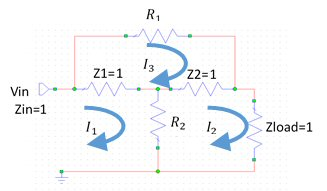# Bridged Tee Fractional Dissipation

Page under construction, come back soon!

New for November 2015: contributed by Terry.  This is a math derivation of the fractional distributions in a bridged-tee attenuator. Below, three current loops are defined. Kirchhoff's Law states that the net current into any node must be zero.In order to greatly simplify the math, we make the following assumptions:

1. All resistances are normalized to Z0
2. Pin is 1 Watt
3. The circuit is designed to be matched.
4. Z1=Z2=Z0=1 by normalization

Pin=1=(I1)^2*Zin ∴ I1=1

G^2≡Pout/Pin... G=I2^2

(1) -I1*1+(I1-I3)*1+(I1-(I2)*R2=0

(2) (I2-I3)*1*(I2*1+((I2-I1)*R2=0

(3) I3*R1+(I3-(I2)*1+(I3-I1)*1=0

Equation (1) becomes:       I3=(1-G)*R2

Equation (2) becomes:       I3=(2+R2)*G-R2

Hence:

I1=1

I2=G

I3=(1-G)*R2

Combining Equations 1 & 2 yields

(4) R2=G/(1-G)

where   G=10^(att/20)         and att is in dB

For R1:  I3=(Vin-Vout)/R1=(I1*1-(I2*1)/R1

(5) I3=(1-G)/R1

Combining Equations 1 & 5 yields:          I3=(1-G)/R1=(1-G)*R2

therefore

(5) R1*R2=1

For dissipations of R1 and R2

(7) PR1=(1-G)^2*R2

PR2=(1-G2)^2/R1

(8) PR1=PR2 because R2=1/R1

For Z1 and Z2 dissipation

PZ1=((I2-I3)^2*1... PZ1=(1-G)^2

PZ2=((I2-I3)*1... PZ2=0

Author : The Other Editor